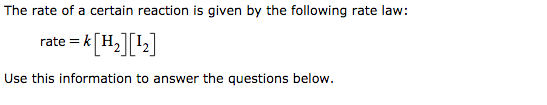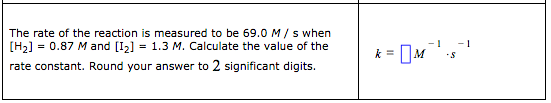# The rate of a certain reaction is given by the following rate law: rate = k[H2][I2] Use this information to answer the questions below. The rate of the reaction is measured to be 69.0 M / s when [H2] = 0.87 and [I2] = 1.3 M. Calculate the value of the rate constant. Round your answer to 2 significant digits.